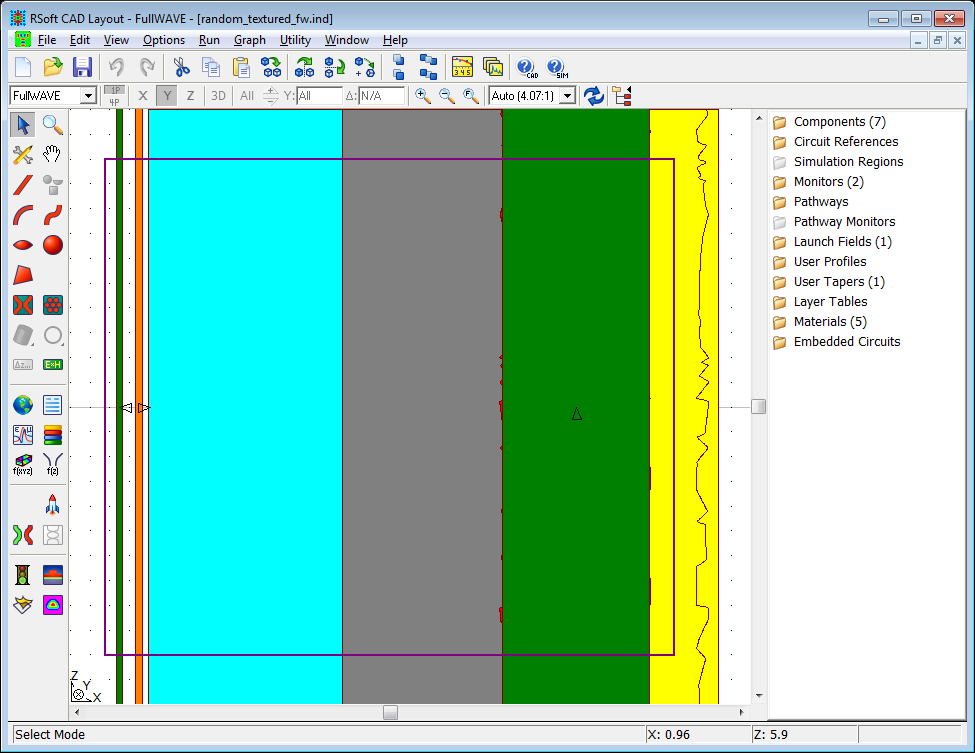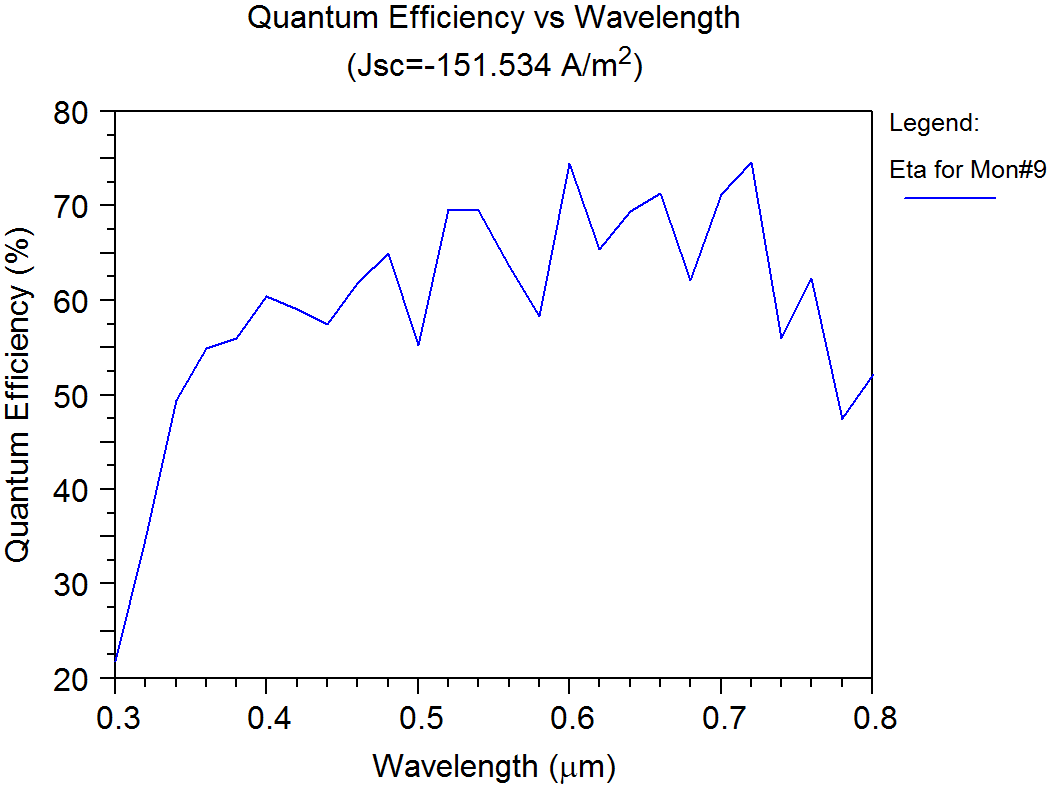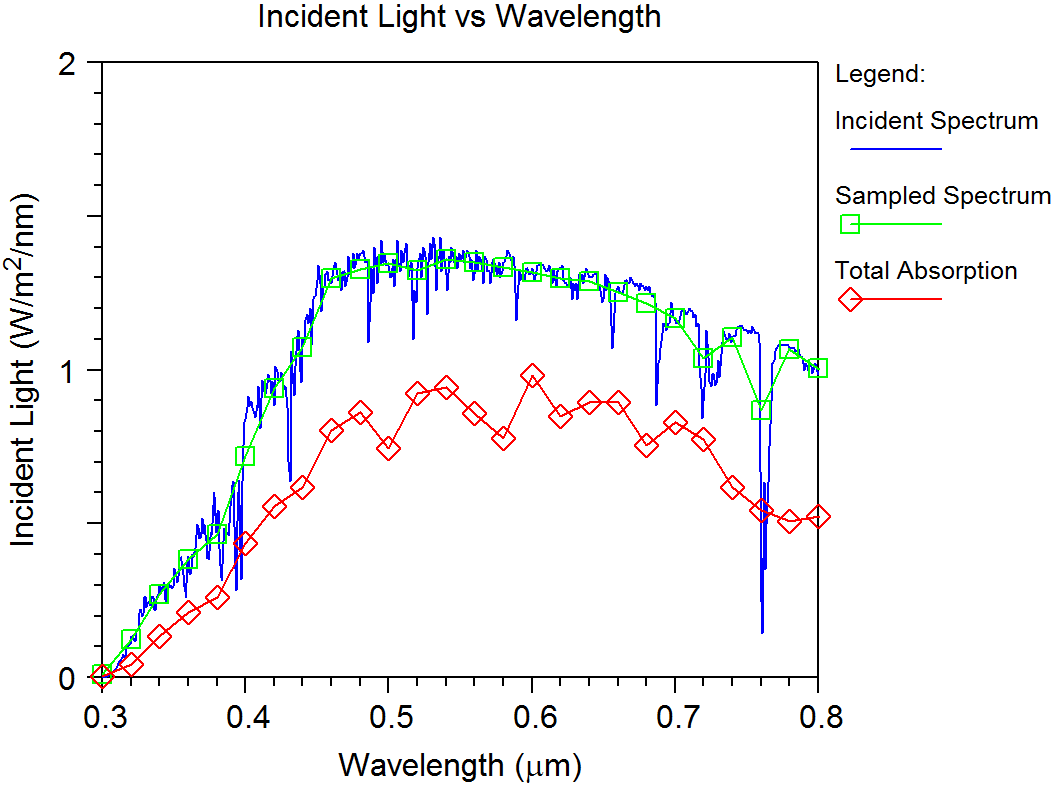# RSoft Application Gallery Note: Modelling Randomly Textured Solar Cells

## Introduction

Randomly textured material interfaces can be used to scatter light within a solar cell and effectively increase the path-length within the structure, therefore increasing the absorption within the silicon region. This example uses RSoft's Solar Cell Utility along with FullWAVE to study the quantum efficiency and absorption spectrum of a randomly-textured solar cell.

## Structure Overview

This solar cell consists of the following layers from front to back: SiO2, SnO2, a thin AZO layer, an amorphous Si absorptive core, AZO, and a silver reflector. Many of the material interfaces are defined to be randomly textured as can be seen in Fig. 1. This is accomplished through the use of width tapers and overlapping segments in the CAD. The structure is assumed to be periodic outside the simulation domain marked in the figure, resulting in a locally random structure that is periodic on a larger scale. Only the absorption within the irregularly-shaped amorphous Si region contributes to the cell efficiency.

The launch field is a plane wave with a TE polarization that is incident along the positive X axis (left to right in Fig. 1).Figure 1. Randomly Textured Solar Cell Schematic

## Calculating the Absorption Spectrum

An optical simulation tool such as RSoft's FullWAVE, which is based on the Finite-Difference Time-Domain algorithm (FDTD), provides a full-vector solution to model the electro-magnetic fields within the structure and to compute the absorption spectrum of the device. Given this absorption spectrum and the incident solar spectrum, the total number of photons absorbed by the solar cell can be computed for a specific wavelength range.

## Using RSoft’s Solar Cell Utility to Compute the Quantum Efficiency

After using an optical model to compute the absorption spectrum of the device, this optical information is then passed down to one of the Solar Cell Utility’s built-in electrical models, which can then compute the cell efficiency. The Solar Cell Utility’s Ideal Diode electrical model uses the ideal diode equation to compute the electrical properties of the device, and can give accurate results if the user has some knowledge about the electrical aspects of the device.

The Absorption spectrum and the Quantum efficiency of the structure shown in Fig. 1 is shown below. The analysis shows that the quantum efficiency of this solar cell is approximately 65%.(a)(b)

Figure 2: The calculation results showing (a) the quantum efficiency, and (b) the various spectra.